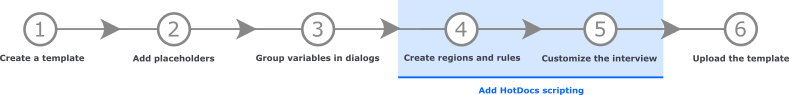# COUNT Function

The COUNT function tells you how many values the template user provided in the interview; it can count the number of repetitions in a repeated dialog, the number of selections in a Multi Select variable, or the number of rows in a Table variable.

The difference between COUNT and COUNTER is that COUNT counts the number of repetitions in a list, while COUNTER gives you the number (index) of the current repetition.

You can use the COUNT function with either a repeated dialog, a Multi Select variable, or a Table variable to insert appropriate language, control interview flow, or otherwise determine a template's logic.

 Function name COUNT Usage model COUNT ( DIALOG,TABLE_VAR,MULT_SELECT_VAR ) Parameters The COUNT functions requires you to replace the model with either a repeated dialog, a Table variable, a Multi Select variable. DIALOG The repeated dialog for which you want to know the number of answers the user has provided. MULT_SELECT_VAR The Multi Select variable for which you want to know the number of options the user has selected. TABLE_VAR The Table variable whose rows you wish to count. Result A number value.

## Example

Suppose you want your template to insert either the plural or singular version of a word. You could use COUNT to do that for you as follows:

```IF COUNT ( items ) = 1
"Item"
ELSE IF COUNT ( items ) > 1
"Items"
END IF ```